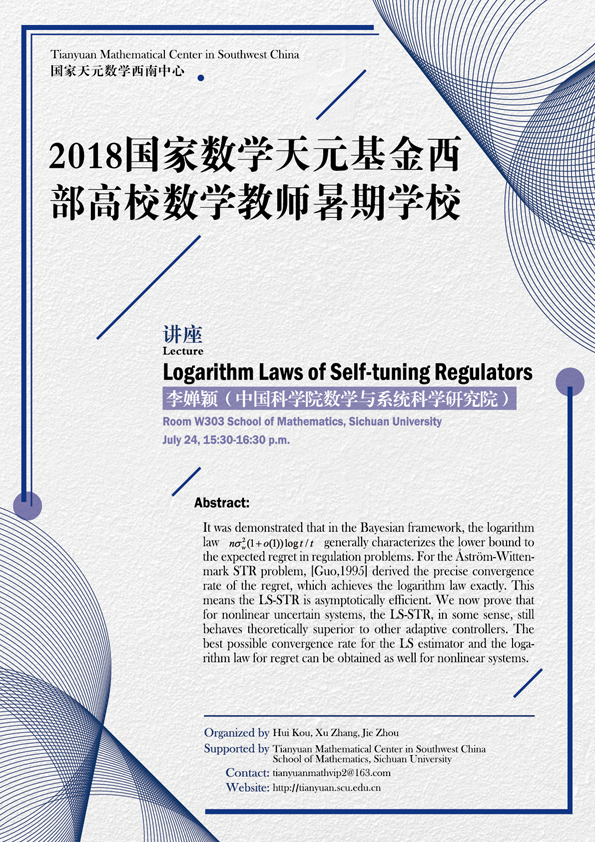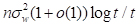Logarithm Laws of Self-tuning Regulators

#### W303  School of Mathematics, Sichuan University#### ABSTRACT

It was demonstrated that in the Bayesian framework, the logarithm lawgenerally characterizes the lower bound to the expected regret in regulation problems. For the Åström-Wittenmark STR problem, [Guo,1995] derived the precise convergence rate of the regret, which achieves the logarithm law exactly. This means the LS-STR is asymptotically efficient. We now prove that for nonlinear uncertain systems, the LS-STR, in some sense, still behaves theoretically superior to other adaptive controllers. The best possible convergence rate for the LS estimator and the logarithm law for regret can be obtained as well for nonlinear systems.

#### ORGANIZERS

Hui Kou (Sichuan University)

Xu Zhang (Sichuan University)

Jie Zhou (Sichuan University)

#### SUPPORTED BY

Tianyuan Mathematical Center in Southwest China

School of Mathematics, Sichuan University